mscroggs.co.uk
mscroggs.co.uksubscribe

# Blog

2017-03-08
This post appeared in issue 05 of Chalkdust. I strongly recommend reading the rest of Chalkdust.
Take a long strip of paper. Fold it in half in the same direction a few times. Unfold it and look at the shape the edge of the paper makes. If you folded the paper $$n$$ times, then the edge will make an order $$n$$ dragon curve, so called because it faintly resembles a dragon. Each of the curves shown on the cover of issue 05 of Chalkdust is an order 10 dragon curve.Top: Folding a strip of paper in half four times leads to an order four dragon curve (after rounding the corners). Bottom: A level 10 dragon curve resembling a dragon.
The dragon curves on the cover show that it is possible to tile the entire plane with copies of dragon curves of the same order. If any readers are looking for an excellent way to tile a bathroom, I recommend getting some dragon curve-shaped tiles made.
An order $$n$$ dragon curve can be made by joining two order $$n-1$$ dragon curves with a 90° angle between their tails. Therefore, by taking the cover's tiling of the plane with order 10 dragon curves, we may join them into pairs to get a tiling with order 11 dragon curves. We could repeat this to get tilings with order 12, 13, and so on... If we were to repeat this ad infinitum we would arrive at the conclusion that an order $$\infty$$ dragon curve will cover the entire plane without crossing itself. In other words, an order $$\infty$$ dragon curve is a space-filling curve.
Like so many other interesting bits of recreational maths, dragon curves were popularised by Martin Gardner in one of his Mathematical Games columns in Scientific American. In this column, it was noted that the endpoints of dragon curves of different orders (all starting at the same point) lie on a logarithmic spiral. This can be seen in the diagram below.The endpoints of dragon curves of order 1 to 10 with a logarithmic spiral passing through them.
Although many of their properties have been known for a long time and are well studied, dragon curves continue to appear in new and interesting places. At last year's Maths Jam conference, Paul Taylor gave a talk about my favourite surprise occurrence of a dragon.
Normally when we write numbers, we write them in base ten, with the digits in the number representing (from right to left) ones, tens, hundreds, thousands, etc. Many readers will be familiar with binary numbers (base two), where the powers of two are used in the place of powers of ten, so the digits represent ones, twos, fours, eights, etc.
In his talk, Paul suggested looking at numbers in base -1+i (where i is the square root of -1; you can find more adventures of i here) using the digits 0 and 1. From right to left, the columns of numbers in this base have values 1, -1+i, -2i, 2+2i, -4, etc. The first 11 numbers in this base are shown below.
 Number in base -1+i Complex number 0 0 1 1 10 -1+i 11 (-1+i)+(1)=i 100 -2i 101 (-2i)+(1)=1-2i 110 (-2i)+(-1+i)=-1-i 111 (-2i)+(-1+i)+(1)=-i 1000 2+2i 1001 (2+2i)+(1)=3+2i 1010 (2+2i)+(-1+i)=1+3i
Complex numbers are often drawn on an Argand diagram: the real part of the number is plotted on the horizontal axis and the imaginary part on the vertical axis. The diagram to the left shows the numbers of ten digits or less in base -1+i on an Argand diagram. The points form an order 10 dragon curve! In fact, plotting numbers of $$n$$ digits or less will draw an order $$n$$ dragon curve.Numbers in base -1+i of ten digits or less plotted on an Argand diagram.
Brilliantly, we may now use known properties of dragon curves to discover properties of base -1+i. A level $$\infty$$ dragon curve covers the entire plane without intersecting itself: therefore every Gaussian integer (a number of the form $$a+\text{i} b$$ where $$a$$ and $$b$$ are integers) has a unique representation in base -1+i. The endpoints of dragon curves lie on a logarithmic spiral: therefore numbers of the form $$(-1+\text{i})^n$$, where $$n$$ is an integer, lie on a logarithmic spiral in the complex plane.
If you'd like to play with some dragon curves, you can download the Python code used to make the pictures here.

### Similar postsDragon curvesHarriss and other spirals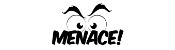MENACE at Manchester Science FestivalThe Mathematical Games of Martin Gardner

Comments in green were written by me. Comments in blue were not written by me.

Allowed HTML tags: <br> <a> <small> <b> <i> <s> <sup> <sub> <u> <spoiler> <ul> <ol> <li>
To prove you are not a spam bot, please type "t" then "h" then "e" then "o" then "r" then "e" then "m" in the box below (case sensitive):
2016-10-08
During my Electromagnetic Field talk this year, I spoke about @mathslogicbot, my Twitter bot that is working its way through the tautologies in propositional calculus. My talk included my conjecture that the number of tautologies of length $$n$$ is an increasing sequence (except when $$n=8$$). After my talk, Henry Segerman suggested that I also look at the number of contradictions of length $$n$$ to look for insights.
A contradiction is the opposite of a tautology: it is a formula that is False for every assignment of truth values to the variables. For example, here are a few contradictions:
$$\neg(a\leftrightarrow a)$$ $$\neg(a\rightarrow a)$$ $$(\neg a\wedge a)$$ $$(\neg a\leftrightarrow a)$$
The first eleven terms of the sequence whose $$n$$th term is the number of contradictions of length $$n$$ are:
$$0, 0, 0, 0, 0, 6, 2, 20, 6, 127, 154$$
This sequence is A277275 on OEIS. A list of contractions can be found here.
For the same reasons as the sequence of tautologies, I would expect this sequence to be increasing. Surprisingly, it is not increasing for small values of $$n$$, but I again conjecture that it is increasing after a certain point.

### Properties of the sequences

There are some properties of the two sequences that we can show. Let $$a(n)$$ be the number of tautolgies of length $$n$$ and let $$b(n)$$ be the number of contradictions of length $$n$$.
First, the number of tautologies and contradictions, $$a(n)+b(n)$$, (A277276) is an increasing sequence. This is due to the facts that $$a(n+1)\geq b(n)$$ and $$b(n+1)\geq a(n)$$, as every tautology of length $$n$$ becomes a contraction of length $$n+1$$ by appending a $$\neg$$ to be start and vice versa.
This implies that for each $$n$$, at most one of $$a$$ and $$b$$ can be decreasing at $$n$$, as if both were decreasing, then $$a+b$$ would be decreasing. Sadly, this doesn't seem to give us a way to prove the conjectures, but it is a small amount of progress towards them.

### Similar postsLogic bot, pt. 2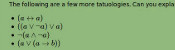Logic botHow OEISbot worksRaspberry Pi weather station

Comments in green were written by me. Comments in blue were not written by me.

Allowed HTML tags: <br> <a> <small> <b> <i> <s> <sup> <sub> <u> <spoiler> <ul> <ol> <li>
To prove you are not a spam bot, please type "regetni" backwards in the box below (case sensitive):
2016-10-06
Thanks to Marc, I noticed that today's date is a palindrome in two different date formats—DMMYY (61016) and DMMYYYY (6102016).
This made me wonder when there will be another date that is palindromic in multiple date formats, so I wrote a Python script to find out.
Turns out there's not too long to wait: 10 July 2017 will be palindromic in two date formats (MDDYY and MDDYYYY). But before that, there's 1 July 2017, which is palindromic in three date formats (YYMMD, YYMD and MDYY). Most exciting of all, however, is 2 February 2020, which is palindromic in 7 different formats!
The next palindromic dates are shown in the following table. It will update as the dates pass.
 $$n$$ Next day with $$\geq n$$ palindromic formats Formats 1 1 September 2019 YYMMD,YYMD,MDYY 2 1 September 2019 YYMMD,YYMD,MDYY 3 1 September 2019 YYMMD,YYMD,MDYY 4 2 February 2020 YYYYMMDD,DDMMYYYY,MMDDYYYY,YYYYMDD,YYMDD,DDMYY,MMDYY 5 2 February 2020 YYYYMMDD,DDMMYYYY,MMDDYYYY,YYYYMDD,YYMDD,DDMYY,MMDYY 6 2 February 2020 YYYYMMDD,DDMMYYYY,MMDDYYYY,YYYYMDD,YYMDD,DDMYY,MMDYY 7 2 February 2020 YYYYMMDD,DDMMYYYY,MMDDYYYY,YYYYMDD,YYMDD,DDMYY,MMDYY
A full list of future palindromic dates can be found here.

### Similar postsDragon curves II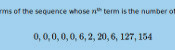Logical contradictionsDragon curvesHow OEISbot works

Comments in green were written by me. Comments in blue were not written by me.

Allowed HTML tags: <br> <a> <small> <b> <i> <s> <sup> <sub> <u> <spoiler> <ul> <ol> <li>
To prove you are not a spam bot, please type "s" then "e" then "g" then "m" then "e" then "n" then "t" in the box below (case sensitive):
2016-03-30
Take a piece of paper. Fold it in half in the same direction many times. Now unfold it. What pattern will the folds make?
I first found this question in one of Martin Gardner's books. At first, you might that the answer will be simple, but if you look at the shapes made for a few folds, you will see otherwise:
The curves formed are called dragon curves as they allegedly look like dragons with smoke rising from their nostrils. I'm not sure I see the resemblance:
As you increase the order of the curve (the number of times the paper was folded), the dragon curve squiggles across more of the plane, while never crossing itself. In fact, if the process was continued forever, an order infinity dragon curve would cover the whole plane, never crossing itself.
This is not the only way to cover a plane with dragon curves: the curves tessellate.When tiled, this picture demonstrates how dragon curves tessellate. For a demonstration, try obtaining infinite lives...
Dragon curves of different orders can also fit together:
To generate digital dragon curves, first notice that an order $$n$$ curve can be made from two order $$n-1$$ curves:
This can easily be seen to be true if you consider folding paper: If you fold a strip of paper in half once, then $$n-1$$ times, each half of the strip will have made an order $$n-1$$ dragon curve. But the whole strip has been folded $$n$$ times, so is an order $$n$$ dragon curve.
Because of this, higher order dragons can be thought of as lots of lower order dragons tiled together. An the infinite dragon curve is actually equivalent to tiling the plane with a infinite number of dragons.
If you would like to create your own dragon curves, you can download the Python code I used to draw them from GitHub. If you are more of a thinker, then you might like to ponder what difference it would make if the folds used to make the dragon were in different directions.

### Similar postsDragon curves IIHarriss and other spirals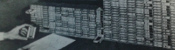MENACE in fiction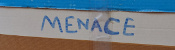Building MENACEs for other games

Comments in green were written by me. Comments in blue were not written by me.

Allowed HTML tags: <br> <a> <small> <b> <i> <s> <sup> <sub> <u> <spoiler> <ul> <ol> <li>
To prove you are not a spam bot, please type "w" then "i" then "d" then "t" then "h" in the box below (case sensitive):
2015-08-29
A few weeks ago, I made OEISbot, a Reddit bot which posts information whenever an OEIS sequence is mentioned.
This post explains how OEISbot works. The full code can be found on GitHub.

### Getting started

OEISbot is made in Python using PRAW (Python Reddit Api Wrapper). PRAW can be installed with:
bash
pip install praw
Before making a bot, you will need to make a Reddit account for your bot, create a Reddit app and obtain API keys. This python script can be used to obtain the necessary keys.
Once you have your API keys saved in your praw.ini file, you are ready to make a bot.

### Writing the bot

First, the necessary imports are made, and test mode is activated if the script is run with test as an argument. We also define an exception that will be used later to kill the script once it makes a comment.
python
import praw
import re
import urllib
import json

import sys
test = False
if len(sys.argv) > 1 and sys.argv == "test":
test = True
print("TEST MODE")

class FoundOne(BaseException):
pass

To prevent OEISbot from posting multiple links to the same sequence in a thread, lists of sequences linked to in each thread can be loaded and saved using the following functions.
python
def save_list(seen, _id):
print(seen)
with open("/home/pi/OEISbot/seen/"+_id, "w"as f:
return json.dump(seen, f)

def open_list(_id):
try:
with open("/home/pi/OEISbot/seen/" + _id) as f:
except:
return []
The following function will search a post for a mention of an OEIS sequence number.
python
def look_for_A(id_, text, url, comment):
seen = open_list(id_)
re_s = re.findall("A([0-9]{6})", text)
re_s += re.findall("oeis\.org/A([0-9]{6})", url)
if test:
print(re_s)
post_me = []
for seq_n in re_s:
if seq_n not in seen:
post_me.append(markup(seq_n))
seen.append(seq_n)
if len(post_me) > 0:
post_me.append(me())
comment(joiner().join(post_me))
save_list(seen, id_)
raise FoundOne
The following function will search a post for a comma-separated list of numbers, then search for it on the OEIS. If there are 14 sequences or less found, it will reply. If it finds a list with no matches on the OEIS, it will message /u/PeteOK, as he likes hearing about possibly new sequences.
python
def look_for_ls(id_, text, comment, link, message):
seen = open_list(id_)
if test:
print(text)
re_s = re.findall("([0-9]+\, *(?:[0-9]+\, *)+[0-9]+)", text)
if len(re_s) > 0:
for terms in ["".join(i.split(" ")) for i in re_s]:
if test:
print(terms)
if terms not in seen:
seen.append(terms)
if test:
print(first10)
if len(first10)>and total <= 14:
if total == 1:
intro = "Your sequence (" + terms \
+ ") looks like the following OEIS sequence."
else:
intro = "Your sequence (" + terms + \
+ ") may be one of the following OEIS sequences."
if total > 4:
intro += " Or, it may be one of the " + str(total-4) \
+ " other sequences listed [here]" \
"(http://oeis.org/search?q=" + terms + ")."
post_me = [intro]
if test:
print(first10)
for seq_n in first10[:4]:
post_me.append(markup(seq_n))
seen.append(seq_n)
post_me.append(me())
comment(joiner().join(post_me))
save_list(seen, id_)
raise FoundOne
elif len(first10) == 0:
post_me = ["I couldn't find your sequence (" + terms \
+ ") in the [OEIS](http://oeis.org). "
message("PeteOK",
"Sequence not in OEIS",
"Hi Peter, I've just found a new sequence (" \
"Please shout at /u/mscroggs to turn the " \
"feature off if its spamming you!")
post_me.append(me())
comment(joiner().join(post_me))
save_list(seen, id_)
raise FoundOne

ls = re.findall("href=(?:'|\")/A([0-9]{6})(?:'|\")", src)
try:
tot = int(re.findall("of ([0-9]+) results found", src))
except:
tot = 0
return ls, tot
The markup function loads the necessary information from OEIS and formats it. Each comment will end with the output of the me function. The ouput of joiner will be used between sequences which are mentioned.
python
def markup(seq_n):
pattern = re.compile("%N (.*?)<", re.DOTALL|re.M)
desc = urllib.urlopen("http://oeis.org/A" + seq_n + "/internal").read()
desc = pattern.findall(desc).strip("\n")
pattern = re.compile("%S (.*?)<", re.DOTALL|re.M)
seq = urllib.urlopen("http://oeis.org/A" + seq_n + "/internal").read()
seq = pattern.findall(seq).strip("\n")
new_com = "[A" + seq_n + "](http://oeis.org/A" + seq_n + "/): "
new_com += desc + "\n\n"
new_com += seq + "..."
return new_com

def me():
return "I am OEISbot. I was programmed by /u/mscroggs. " \
"[How I work](http://mscroggs.co.uk/blog/20). " \
"You can test me and suggest new features at /r/TestingOEISbot/."

def joiner():
return "\n\n- - - -\n\n"
Next, OEISbot logs into Reddit.
python
= praw.Reddit("OEIS link and description poster by /u/mscroggs.")

access_i = r.refresh_access_information(refresh_token=r.refresh_token)
r.set_access_credentials(**access_i)

auth = r.get_me()
The subs which OEISbot will search through are listed. I have used all the math(s) subs which I know about, as these will be the ones mentioning sequences.
python
subs = ["TestingOEISbot","math","mathpuzzles","casualmath","theydidthemath",
"learnmath","mathbooks","cheatatmathhomework","matheducation",
"recreationalmath","OEIS","mathclubs","maths"]
if test:
subs = ["TestingOEISbot"]
For each sub OEISbot is monitoring, the hottest 10 posts are searched through for mentions of sequences. If a mention is found, a reply is generated and posted, then the FoundOne exception will be raised to end the code.
python
try:
for sub in subs:
print(sub)
subreddit = r.get_subreddit(sub)
for submission in subreddit.get_hot(limit = 10):
if test:
print(submission.title)
look_for_A(submission.id,
submission.title + "|" + submission.selftext,
submission.url,
look_for_ls(submission.id,
submission.title + "|" + submission.selftext,
submission.url,
r.send_message)

and comment.author is not None
and comment.author.name != "OEISbot" ):
look_for_A(submission.id,
re.sub("$[^$]*\]$$[^$$*]\)","",comment.body),
comment.body,
look_for_ls(submission.id,
re.sub("$[^$]*\]$$[^$$*]\)","",comment.body),
submission.url,
r.send_message)

except FoundOne:
pass

### Running the code

I put this script on a Raspberry Pi which runs it every 10 minutes (to prevent OEISbot from getting refusals for posting too often). This is achieved with a cron job.
bash
*/10 * * * * python /path/to/bot.py

The full OEISbot code is available on GitHub. Feel free to use it as a starting point to make your own bot! If your bot is successful, let me know about it in the comments below or on Twitter.

### Similar postsLogic botRaspberry Pi weather stationLogical contradictionsLogic bot, pt. 2

Comments in green were written by me. Comments in blue were not written by me.